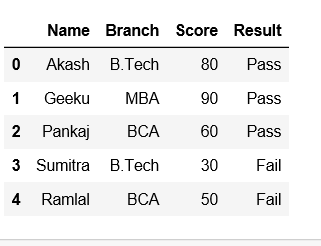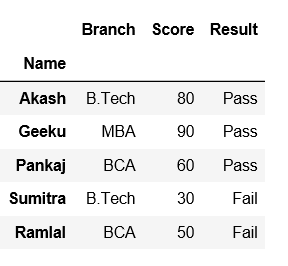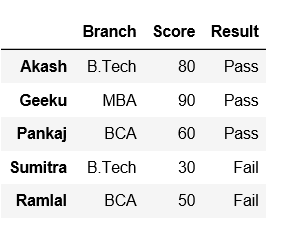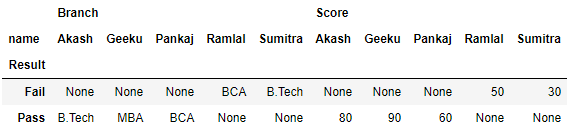# Convert a column to row name/index in Pandas

Pandas provide a convenient way to handle data and its transformation. Let’s see how can we convert a column to row name/index in Pandas.

Create a dataframe first with dict of lists.

## Python3

 `# importing pandas as pd` `import` `pandas as pd`   `# Creating a dict of lists ` `data ``=` `{``'Name'``:[``"Akash"``, ``"Geeku"``, ``"Pankaj"``, ``"Sumitra"``,``"Ramlal"``],` `       ``'Branch'``:[``"B.Tech"``, ``"MBA"``, ``"BCA"``, ``"B.Tech"``, ``"BCA"``],` `       ``'Score'``:[``"80"``,``"90"``,``"60"``, ``"30"``, ``"50"``],` `       ``'Result'``: [``"Pass"``,``"Pass"``,``"Pass"``,``"Fail"``,``"Fail"``]}`   `# creating a dataframe ` `df ``=` `pd.DataFrame(data)` ` `  `df`

Output:Method #1: Using set_index() method.

## Python3

 `# importing pandas as pd` `import` `pandas as pd`   `# Creating a dict of lists` `data ``=` `{``'Name'``:[``"Akash"``, ``"Geeku"``, ``"Pankaj"``, ``"Sumitra"``,``"Ramlal"``],` `       ``'Branch'``:[``"B.Tech"``, ``"MBA"``, ``"BCA"``, ``"B.Tech"``, ``"BCA"``],` `       ``'Score'``:[``"80"``,``"90"``,``"60"``, ``"30"``, ``"50"``],` `       ``'Result'``: [``"Pass"``,``"Pass"``,``"Pass"``,``"Fail"``,``"Fail"``]}`   `# Creating a dataframe` `df ``=` `pd.DataFrame(data)`   `# Using set_index() method on 'Name' column` `df ``=` `df.set_index(``'Name'``)`   `df`

Output:Now, set index name as None.

## Python3

 `# set the index to 'None' via its name property` `df.index.names ``=` `[``None``]`   `df`

Output:Method #2: Using pivot() method.
In order to convert a column to row name/index in dataframe, Pandas has a built-in function Pivot.
Now, let’s say we want Result to be the rows/index, and columns be name in our dataframe, to achieve this pandas has provided a method called Pivot. Let us see how it works,

## Python3

 `# importing pandas as pd` `import` `pandas as pd`   `# Creating a dict of lists` `data ``=` `{``'name'``:[``"Akash"``, ``"Geeku"``, ``"Pankaj"``, ``"Sumitra"``, ``"Ramlal"``],` `       ``'Branch'``:[``"B.Tech"``, ``"MBA"``, ``"BCA"``, ``"B.Tech"``, ``"BCA"``],` `       ``'Score'``:[``"80"``, ``"90"``, ``"60"``, ``"30"``, ``"50"``],` `       ``'Result'``: [``"Pass"``, ``"Pass"``, ``"Pass"``, ``"Fail"``, ``"Fail"``]}`   `df ``=` `pd.DataFrame(data)`   `# pivoting the dataframe` `df.pivot(index ``=``'Result'``, columns ``=``'name'``)`   `df`

Output:Whether you're preparing for your first job interview or aiming to upskill in this ever-evolving tech landscape, GeeksforGeeks Courses are your key to success. We provide top-quality content at affordable prices, all geared towards accelerating your growth in a time-bound manner. Join the millions we've already empowered, and we're here to do the same for you. Don't miss out - check it out now!

Previous
Next